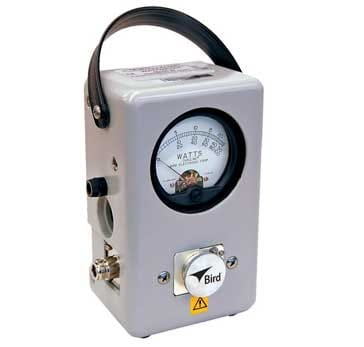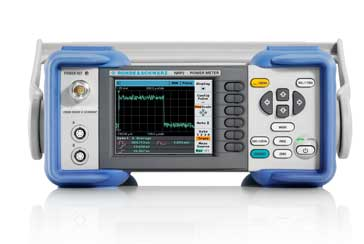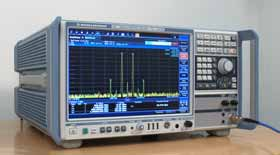# What is an RF & Microwave Power Meter / Wattmeter: tutorial

## RF power meters or wattmeters are used for measuring power in RF circuits: power can be measured in a variety of ways dependent upon the application.

RF & microwave power meter Includes:
RF power meter     PEP, average, & pulse power     Power measurement techniques     In-line power meter     Absorption-power meters & sensors

In many areas of RF development, design, test, service repair and field service, RF and microwave power meters or wattmeters are needed.

RF power is a key parameter for many applications, and as a result, RF power meters are needed.

RF power measurement can be undertaken in a variety of ways, each having its own characteristics, advantages and disadvantages. It is necessary to choose the right form of power measurement for any particular application. In this way the power measurement results will reveal the required data and enable the system to be tested in the correct manner.

While RF power measurement may be thought is as simply using an RF power meter or a microwave power meter, this is not just the case. Knowing the different measurement techniques, understanding the different types of RF power meter, and ways in which the errors in RF and microwave power measurements can be minimised are all key to making good RF power readings.

## Types of RF and microwave power meter

There are a number of ways in which RF power (including microwave power) can be measured. As a result there are different classes of test instrument that can be used for RF power measurement:

• In-line RF power meters:   These RF power meters take a sample of the power flowing along a feed-line and use this to indicate the power level. These inline RF power meters are used on live systems, such as radio transmitters as a check of the outgoing power. They are normally directional and can be used to check the power travelling in either direction.The iconic Bird 43 Thuline ® Wattmeter Measurements made by these RF power meters are frequency insensitive - they measure the total power entering them regardless of frequency (within the overall frequency limitations of the instrument).

Read more about . . . . Inline Wattmeter / Power Meter.
• Absorptive RF power meters:   As their name implies, these RF power meters absorb the power they measure. Simple meters can be analogue types that are formed in a single unit consisting of an RF load, the sensing diodes and an analogue meter. These are adequate for a number of applications, but can be limited in their performance.

The more advanced types utilise an external power sensor that can be connected close to the power source, and these power sensors may be one of a variety of types. This generates a signal proportional to the power level entering the sensor. The sensor signal is coupled to the main instrument within the overall RF power meter to process the results and display the reading.

Measurements made by absorptive RF power meters are frequency insensitive - they measure the total power entering them regardless of frequency (within the overall frequency limitations of the instrument).

The absorptive RF power meters with external sensors typically have either digital readouts, or many these days provide a display on which the result can be seen in the most convenient format. For operation, an analogue voltage is generated within the power sensor or power head and this is fed into the main RF power meter unit. With high levels of digital signal processing available these days, many RF power meters contain significant levels of processing and this can enable a variety of signal types to be measured.Typical absorption RF / microwave power meter that uses an external sensor When selecting an RF power meter or a microwave power meter, it is important to select the correct type of power sensor. There are a number of different types of power sensor, and these are suited to different types of RF power measurement. Some types of RF power sensor are suited to make measurements of average power, whereas others can make measurements of pulse power or peak envelope power.

• Spectrum analysers and other instruments:   Instruments such as spectrum analysers have power measurement capabilities within them. These instruments are able to measure the RF power level on a particular frequency, but cannot measure the total power entering on all frequencies. Spectrum analyser RF power measurements used not to be accurate, but with the improvements in their technology, the RF power measurements have far greater levels of accuracy.Spectrum analyzer showing signals for which the power can be measured As might be expected, the power measurements made with spectrum analyzers are frequency sensitive and it is possible to measure the power of a signal on a particular frequency. This can be useful when requiring power measurements for a fundamental frequency or harmonics or other spurious signals.

### Note on the Spectrum Analyzer:

Spectrum analyzers analyzers make measurements of signals in the frequency domain, displaying signal levels against frequency. As such spectrum analyzers are particularly appropriate for many areas like RF design, service and repair, where the levels of signals on different frequencies need to be viewed.

Each type of RF power meter or wattmeter is used under different circumstances. However the absorptive RF power meter is the most widely used for accurate laboratory measurements. The through-line power meters tend to be used more for field applications.

## Units for RF and microwave power measurements

Power is a measure of energy per unit time and it is typically measured in watts - this is a energy transfer at the rate of one Joule per second.

Although the watt is the base measure, often this is preceded by a multiplier as power levels can extend over a vast range. Levels of kilowatts (103 watts), or even megawatts (106 watts) are used in some large power installations, whereas other applications have much lower levels - milliwatts (10-3 watts), or microwatts (10-6 watts) may be found.

In some instances power may be specified in terms of dBW or dBm. These use a logarithmic decibel scale but related to a given power level.

In itself a decibel is not an absolute level. It is purely a comparison between two levels, and on its own it cannot be used to measure an absolute level. The quantities of dBm and dBW are the most commonly used.

### Note on dBm & dBW:

Many power levels are expressed in terms of dBm and dBW. As a decibel is a ratio, these are power levels expressed in terms of decibels relative a milliwatt and a watt respectively.